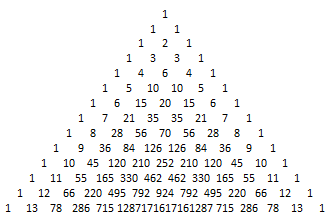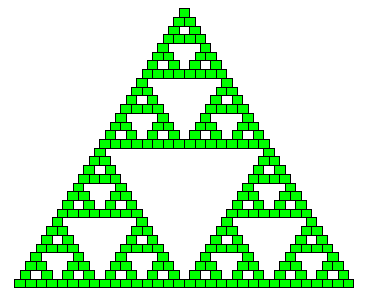# Tag: fractals

Entries for tag "fractals", ordered from most recent. Entry count: 9.

Warning! Some information on this page is older than 5 years now. I keep it for reference, but it probably doesn't reflect my current knowledge and beliefs.

# Rhipsalis - a Fractal Plant

Sun
29
Sep 2013

Fractals appear in nature. Probably the most spectacular example is Romanesco Broccoli. But there is also an interesting plant that looks like very regular L-system. I was wondering what is it since I first saw it in my friend's house. Now I know it's called Rhipsalis and it's a kind of cactus. Here is my small one:# Coloring of Fractal Flame

Wed
23
Dec 2009

My next step in Fractal Flames rendering was to enhance the way I give colors to pixels on the final texture. As positions of the points iterated through the function system are discretized to a 3D matrix, but before it's processed into the final texture, I've introduced a choice of matrix format (inspired by texture formats in DirectX).

The simplest one is MATRIX_FORMAT_DENSITY, as it has only one component per cell - a density, equal to number of points that hit this particular cell. No coloring is done here, so each pixel is just white and there is only density influencing its brightness through tone mapping.

Second format is MATRIX_FORMAT_COLOR_DENSITY. It works similar to what is described in The Fractal Flame Algorithm paper. Each processed point carries a "color" with it, but this "color" is just a scalar value in range 0..1. Every possible transform (transforms are chosen in random manner according to their probabilities) pushes the color towards its "desired" value, defined for each transform. Current point color is added to the matrix cell so that final color is an average of colors of all points that hit this cell.

To visualize this scalar "color", it's nice to process it through some lookup table, gradient, palette or however you call it. I seems easy to generate or hardcode one, but I prefer to rely on existing gradients prepared by more talented people, so I've implemented reading of two gradient formats so far: gradient from a single-row texture or from a "cmap.UGR" file shipped with Apophysis (nice, free Windows application that render fractal flames according to the paper mentioned above). I'm also planning to support "ggr" files with gradients from GIMP, but it's far more sophisticated and powerful format.And finally there is the third way of coloring fractal flames that seems most intuitive to me - MATRIX_FORMAT_RGB_DENSITY. Here, full three color components are carried with points and saved to the matrix. Each transform pushes the point color towards its own "desired" RGB value. Thanks to this, each transform influences not only shape, but also color of the fractal in direct and observable way (like the scaling transform here, that shrinks points to the center, which is red).# I Render Fractal Flames

Sun
20
Dec 2009

Continuing my learning/research of fractals, today I've finally managed to render something that looks like Fractal Flame. AFAIK the most important paper on this subject is The Fractal Flame Algorithm by Scott Draves and Erik Reckase. According to this document, I've first added to my program the ability to use colors, so each point, as it has the position iteratively processed by some functions, also carries current color. Now I am able to render colourful IFS fractals:

Implementing logarithmic tone mapping and gamma correction significantly improved quality. Colors can help visualising internal structure of a fractal:

Next I've coded (almost) all functions mentioned in this paper, so now not only affine transforms are possible in my program. This allows me to mix traditional fractals with new functionality:

And finally to render something that looks like famous Fractal Flames. But it's not easy to achieve this. Modifying numeric parameters in a text file is not convenient, and even if I had an editor with real-time preview, it's not obvious what parameters give what results and whether they will degenerate to something like a single point. There are simply too many degrees of freedom :) If we had a continuous function Beauty(Params), we could use some optimization algorithms to find and render its local extrema :) Anyway, here are my first renders (more in Fractal Flames Gallery).

# Further Experiments with Fractals

Sun
13
Dec 2009

Today I woke up with an idea of inventing my own simple IFS fractal with four pieces sheared or rotated to form a hole in the center. I must admin that effects are not impressive :) What I've learned is that it's difficult to imagine how overall fractal will generally look like when you design affine transforms for a single step that will be processed recursively. But anyway I'll show my results, with single step on the left and final render on the right:

Shear transform which unexpectedly formed round shapes:Rotation transform with boundaries exceeding -1..1 range:Rotation transform bound in range (-1..1, -1..1):# Rendering Mandelbrot and Julia Fractal

Thu
10
Dec 2009

I've been playing recently with Mandelbrot and Julia sets. These are fractals rendered in the completely different way that my last experiments with IFS (Iterated Function Systems). In IFS I had a set of points with given (x,y) positions and I've been transforming their positions iteratively by some functions before I rendered them. I'll blog more about IFS another time. Rendering Mandelbrot and Julia fractals is more like writing a pixel shader - for every pixel on the screen with its (x,y) coordinates we do some computations to calculate final color. I didn't extend my framework to render such things yet, but instead I've been doing my experiments in EvalDraw (where I can freely pan and zoom the view) and AMD RenderMonkey (where I can write pixel shaders executed on GPU).

Complex Numbers for Game Developers :) Fractal is a mathematical concept and so there is lots of hardcore math involved in the foundation of these beautiful objects. But fortunately we don't have to understand all that stuff to be able to render them. Mandelbrot and Julia fractals are created using complex numbers, but for us they can be thought of just as float2/D3DXVECTOR2 2-component (x,y) vectors with some operations defined:

• Addition: (x1, y1) + (x2, y2) = (x1+x2, y1+y2)
• Muliplication: (x1, y1) * (x2, y2) = (x1*x2 - y1*y2, y1*x2 + x1*y2)
• Square: (x, y)^2 = (x, y) * (x, y) = (x*x - y*y, 2*x*y)

That's actually all we need to render Mandelbrot and Julia fractals. The general formula for both of them is: z = z^2 + c where z and c are complex numbers, c is a constant and z is recalculated many times through this function. It was a mystery for me for a long time how to apply this formula. Now it turned out that when we calculate it many times for each pixel with staring z_start = (0, 0) and c dependant on pixel position in range (-2..2, -2..2) and draw all the pixels for which z is bounded and do not go to infinity, we get the shape of the Mandelbrot fractal. Of course we are not going to iterate this function inifinite times in our computers like mathematicians do in their heads, so instead we do it several times and treat all pixels for which length(z_final) < 2 as being "inside" the shape. Here is a draft made in EvalDraw. Unfortunately we meet some limitations of floating point numbers here, like limited precision and infinites and that's why the space outside is white.To render Julia set we do something similar, but this time we use pixel position as starting z and set c to some constant, like (-0.1, 0.7). Different constants make different images.

A question arises about how to render a fractal - how to map a complex number to pixel color? The algorithm I like the most is called Escape Time Algorith and it goes like this: recalculate z for several iterations, but only while the length of z=(x,y) vector is less than 2 (that is zx*zx + zy*zy < 2*2). If the loop reached the iteration count limit, this pixel is inside the fractal - draw in in black. If not, then color of this pixel depends on number of iterations done.

Here are my implementations of these fractals in EvalDraw and RenderMonkey. They are very simple so the whole source code is visible on the screenshots. You can also download the code here: Fractals_for_RenderMonkey.rfx, Mandelbrot_for_EvalDraw_1.kc, Julia_for_EvalDraw_1.kc, Multibrot_for_EvalDraw_1.kc. You can find more screenshots in my fractals gallery.

Some variations of these fractals have also been discovered. For example when we do absolute value of z components before every iteration in Mandelbrot fractal, we get an interesting shape called Burning Ship.

Alternatively, if we raise z to any real power instead of 2, we make Mandelbrot fractal "unfolding", having more and more "bulbs" as the exponent grows. It is called Multibrot set. Raising a complex number to power with any real exponent is a little bit more complicated, as it has to use polar form (HLSL-like pseudocode here):

```float2 ComplexPow(float2 v, float n)
{
float len = sqrt(x*x + y*y); // Standard 2D vector length
float n_fi = n * atan2(y, x);
return pow(len, n) * float2( cos(n_fi), sin(n_fi) ); // scalar * vector
}```

# New Fractal Renders

Sun
06
Dec 2009

Continuing playing with fractals, I've improved my experiment framework so now I can render any IFS (Iterated Function System) fractals described by a set of affine transformations with subpixel quality. Here are my today renders (these with colors are processed with GIMP). You can find more in my Gallery / Fractals.Where do I get information from? There is a great website with ready formulas for each of these fractals: Classic Iterated Function Systems by Larry Riddle from Agnes Scott College.

# Fractals, IFS and a Fern

Sat
14
Nov 2009

Today I've started playing with fractals. Doing such experiments is now easy as I have in my home framework a whole bunch of useful functions like exposing structure fields into a property window or executing computational/IO tasks on the separate thread in the background. My first effect is Barnsley's fern, coded according to the algorithm from Wikipedia.# Properties of Pascal Triangle

Thu
12
Nov 2009

Pascal Triangle is a mathematical object that looks like triangle with numbers arranged the way like bricks in the wall. Each next row has one more number, ones on both sides and every inner number is the sum of two numbers above it. It can span infinitely.Despite simple algorithm this triangle has some interesting properties. First, if you draw only odd numbers, you get a fractal - Sierpinski Triangle.Second, graph of numbers in each row resembles Gaussian distribution function. The lower row you take from the triangle (containing more numbers), the more precise graph you get.I've made these images with Octave - a free Matlab alternative. I like it and I think concepts behind this Matlab programming language can be quite useful for doing computations, but doing graphics is painfully slow. Whole seconds to draw several dozens of texts or rectangles? WTF?!